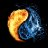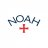# Onset Trend Detector Indicator for ThinkorSwim#### BenTen

Staff
VIP
Onset Trend Detector is an indicator for ThinkorSwim that will give buy and sell signals. This script was developed by John F. Ehlers.> Configure two lower studies

>  cutoffLength = 20 / k = 0.8

>  cutoffLength = 20 / k = 0.4

> Rules:

>  Buy when the first 30-period QT with K = 0.8 crosses 0 from below

>  Sell the position when the second 30-period QT with K = 0.4 crosses 0 from above

thinkScript Code
Rich (BB code):
``````declare lower;

input price = close;
input cutoffLength = 10;
input k = .8;

def alpha1 = (Cos(Sqrt(2) * Double.Pi / 100) + Sin (Sqrt(2) * Double.Pi / 100) - 1) / Cos(Sqrt(2) * Double.Pi / 100);
def highpass = if IsNaN(price + price + price) then highpass else Sqr(1 - alpha1 / 2) * (price - 2 * price + price) + 2 * (1 - alpha1) * highpass - Sqr(1 - alpha1) * highpass;
def filt = reference EhlersSuperSmootherFilter(highpass, cutoffLength);
def peak = if AbsValue(filt) > peak * .991 then AbsValue(filt) else peak * .991;
plot NormRoofingFilter = filt / peak;
plot Quotient = (NormRoofingFilter + k) / (k * NormRoofingFilter + 1);
plot ZeroLine = 0;

NormRoofingFilter.SetDefaultColor(GetColor(1));
Quotient.SetDefaultColor(GetColor(2));
ZeroLine.SetDefaultColor(GetColor(7));``````

https://tos.mx/aQbyVg

Last edited:
Z

##### New member
How do I get the bundles on my chart?

Last edited:#### BenTen

Staff
VIP
@zwarriortrader What do you mean by bundle?

Last edited:
Z

##### New member
I am sorry the bubbles

Last edited:#### BenTen

Staff
VIP
@zwarriortrader That's the Supertrend indicator. You can get it here.

Last edited:4 & 20 Period Historical Volatility - Reversals and Trend Exhaustion Indicators 0Super Trend + 9 ema + VWAP Questions 3